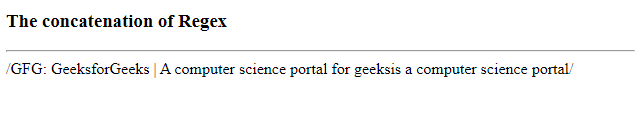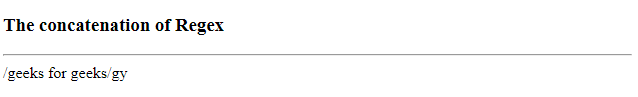# How to concatenate regex literals in JavaScript ?

• Last Updated : 05 Jun, 2020

Regex is a sequence of pattern that is used for matching with a pattern. While searching for data in a text, the search pattern is described for what we are searching for. It can be a single character or a more complex pattern. It can be used to perform all types of text searches. Regex has its own static and instance properties.

Syntax:

```/pattern/modifiers
```

Example: A regular expression.

`/gfg/g`

Where,

• gfg is a pattern (to be used in a search).
• g is a modifier (modifies the search to be case-insensitive).

The concatenation of Regex in the programming world can be understood as combining text patterns
to obtain a new text pattern, such as “Hello” + “World” is /HelloWorld/. Whenever RegExp() is called, it creates a new RegExp object.

Example 1: This example creating an expression without actually using the Regex literal syntax. This allows you to make arbitrary string manipulation before it becomes a Regex object.

 ` ` `<``html``> ` ` `  `<``head``> ` `    ``<``meta` `name``=``"viewport"` `content``= ` `        ``"width=device-width,initial-scale=1.0"` `/> ` ` `  `    ``<``title``> ` `        ``Concatenation of Regex ` `    `` ` ` `  ` ` ` `  `<``body``> ` `    ``<``h3``>The concatenation of Regex ` `    ``<``hr``> ` `     `  `    ``<``script``> ` `        ``function gfg() { ` `            ``var segment_part = " GeeksforGeeks |" ` `                ``+ " A computer science portal for geeks"; ` ` `  `            ``var pattern = new RegExp("GFG:" +  ` `                    ``/*comment here */ ` `                    ``segment_part +  ` `                    ``/* that was defined just now */ ` `                    ``"is a computer science portal"); ` ` `  `            ``document.write(pattern); ` `        ``} ` `        ``gfg(); ` `    `` ` ` ` ` `  ` `

Output:Example 2: If you have two Regex literals, you can concatenate them using a technique where it removes duplicates, but keep the unique values in order, joining both the regex literals.

Example: /hello/y + / world/g would be /hello world/gy

 ` ` `<``html``> ` ` `  `<``head``> ` `    ``<``meta` `name``=``"viewport"` `content``= ` `        ``"width=device-width, initial-scale=1.0"``/> ` ` `  `    ``<``title``> ` `        ``Concatenation of Regex ` `    `` ` ` ` ` `  `<``body``> ` `    ``<``h3``>Concatenation of Regex ` `    ``<``hr``> ` `    ``<``script``> ` `        ``function gfg() { ` `            ``var regex1 = /geeks/g; ` `            ``var regex2 = / for geeks/y; ` `            ``var flags = (regex1.flags +  ` `                ``regex2.flags).split("") ` `                    ``.sort().join("") ` `                     `  `                    ``.replace(/(.)(?=.*\1)/g, ""); ` `            ``var regex3 = new RegExp(regex1.source ` `                        ``+ regex2.source, flags); ` `                         `  `            ``document.write(regex3); ` `        ``} ` `        ``gfg(); ` `    `` ` ` ` ` `  ` `

Output:My Personal Notes arrow_drop_up
Recommended Articles
Page :